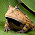### Embedding Python in C++: converting C++ vectors to numpy arrays, and plotting C++ vector contents using matplotlib

Edit: A comment on StackOverflow from user4815162342 gave a helpful suggestion:
You really should look into using PyArray_SimpleNewFromData, as the OP proposed in the question. For larger vectors it is very important to avoid creating a Python list or tuple, because they require all of their elements to be Python objects, which is very memory- and CPU-inefficient for large vectors.

-----------------------------------------------

I haven't found a plotting library for C++ that I like as much as Python's matplotlib, but the prospect of writing data calculated in a C++ program to a file and then reading it into a Python program for plotting was not appealing. Fortunately, there's a Python/C API that enables Python to be embedded into C/C++.

I managed to cobble together code that makes two C++ vectors, converts them to Python tuples, passes them to Python, converts them to NumPy arrays, then plots them using matplotlib. There is probably a better way that folks with more experience can provide, but this seems to work.

Credit goes to these pages for helping me cobble it together:

A Makefile along with C++ and Python code can be found at my Github, but here are some of the highlights.

Here is a bit of the important stuff from the .cpp file:
```     //Make some vectors containing the data
static const double xarr[] = {1,2,3,4,5,6,7,8,9,10,11,12,13,14};
std::vector<double> xvec (xarr, xarr + sizeof(xarr) / sizeof(xarr) );
static const double yarr[] = {0,0,1,1,0,0,2,2,0,0,1,1,0,0};
std::vector<double> yvec (yarr, yarr + sizeof(yarr) / sizeof(yarr) );

//Transfer the C++ vector to a python tuple
pXVec = PyTuple_New(xvec.size());
for (i = 0; i < xvec.size(); ++i) {
pValue = PyFloat_FromDouble(xvec[i]);
if (!pValue) {
Py_DECREF(pXVec);
Py_DECREF(pModule);
fprintf(stderr, "Cannot convert array value\n");
return 1;
}
PyTuple_SetItem(pXVec, i, pValue);
}

//Transfer the other C++ vector to a python tuple
pYVec = PyTuple_New(yvec.size());
for (i = 0; i < yvec.size(); ++i) {
pValue = PyFloat_FromDouble(yvec[i]);
if (!pValue) {
Py_DECREF(pYVec);
Py_DECREF(pModule);
fprintf(stderr, "Cannot convert array value\n");
return 1;
}
PyTuple_SetItem(pYVec, i, pValue); //
}

//Set the argument tuple to contain the two input tuples
PyTuple_SetItem(pArgTuple, 0, pXVec);
PyTuple_SetItem(pArgTuple, 1, pYVec);

//Call the python function
pValue = PyObject_CallObject(pFunc, pArgTuple);
```
Here's the entire .py file:
```def plotStdVectors(x, y):
import numpy as np
import matplotlib.pyplot as plt
print "Printing from Python in plotStdVectors()"
print x
print y
x = np.fromiter(x, dtype = np.float)
y = np.fromiter(y, dtype = np.float)
print x
print y
plt.plot(x, y)
plt.show()
return 0
```
And, after compiling with the Makefile (which is for Ubuntu 12.10 using the system's default Python installation), can be run with:
```\$ ./testEmbed pythonToEmbed plotStdVectors
Hello from main
Hello from runPython()
Printing from Python in plotStdVectors()
(1.0, 2.0, 3.0, 4.0, 5.0, 6.0, 7.0, 8.0, 9.0, 10.0, 11.0, 12.0, 13.0, 14.0)
(0.0, 0.0, 1.0, 1.0, 0.0, 0.0, 2.0, 2.0, 0.0, 0.0, 1.0, 1.0, 0.0, 0.0)
[  1.   2.   3.   4.   5.   6.   7.   8.   9.  10.  11.  12.  13.  14.]
[ 0.  0.  1.  1.  0.  0.  2.  2.  0.  0.  1.  1.  0.  0.]
Result of call: 0
Program finished
```
And the plot:

1.I found this example extremely useful!! So much so that I was finally able to figure out how to hook up MPL directly with my C++ code. I can't get the call to PyArray_SimpleNewFromData to work though. Any chance you had success?

1.I'm glad it worked for you! I haven't gone back to try PyArray_SimpleNewFromData since this worked for my use case; it sounds like PyArray_SimpleNewFromData is the better way to implement it though, so if you have any success with it, please share!